### Differential Evolution (DE)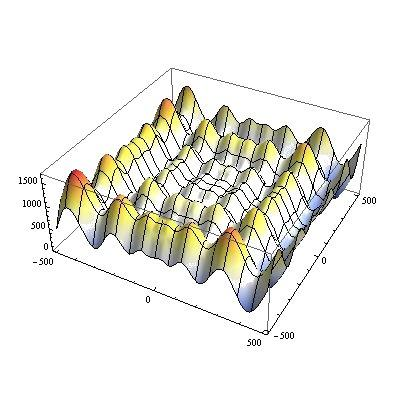## Introduction

xlOptimizer fully implements Differential Evolution (DE), a relatively new stochastic method which has attracted the attention of the scientific community. DE was introduced by Storn and Price  and has approximately the same age as PSO. An early version was initially conceived under the term “Genetic Annealing” and published in a programmer’s magazine . The DE algorithm is extremely simple; the uncondensed C-style pseudocode of the algorithm spans less than 25 lines .

## How does it work?

Differential evolution bears no natural paradigm, i.e. it is not biologically inspired. It is an evolutionary algorithm which evolves a population of possible solutions. Basically, DE adds the weighted difference between two population members to a third member. This simple idea has proved to be an extremely effective method of optimization.

## Mathematical formulation

According to the classic version of DE, a population of p individuals is randomly dispersed within the design space. The population is denoted as Px,g, as follows: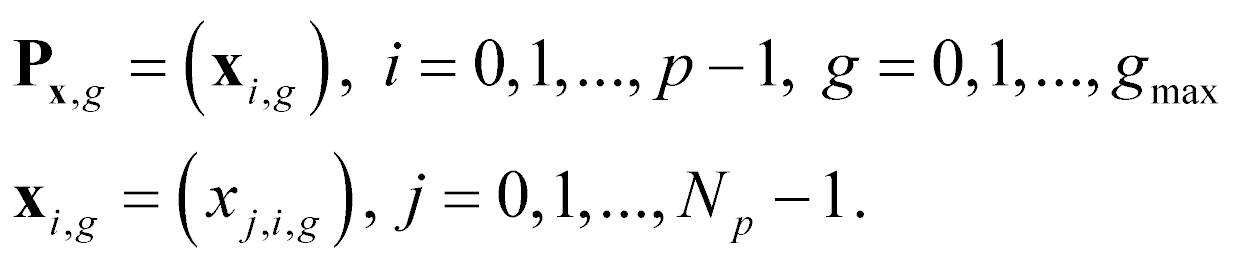where, Px,g= array of p vectors (individuals), xi,g= Np–dimensional vector representing a solution, i=index for vectors, g=index for generations, j=index for design variables, gmax=maximum number of generations and the parentheses indicate an array.

At each evolutionary step (generation), a mutated population Pv,g is formed based on the current population Px,g, as follows: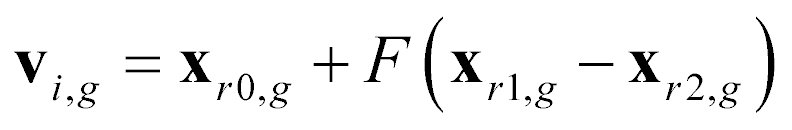where, r0, r1, r2 are random indices in {0,1,…,p-1} and F is a scalar DE algorithm parameter. Next, a trial population Pu,g is formed, as follows: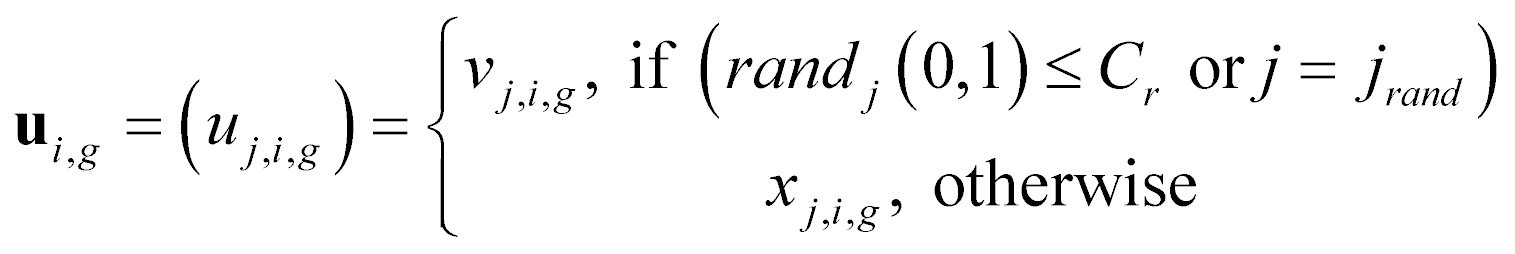where, randj is a random number with uniform distribution in (0,1) that is sampled anew each time, jrand is a random index in {0,1,…,p-1} that ensures that at least one design variable will originate from the mutant vector, and Cr is a scalar DE algorithm parameter in the range (0,1]. The final step is the selection criterion, which is greedy: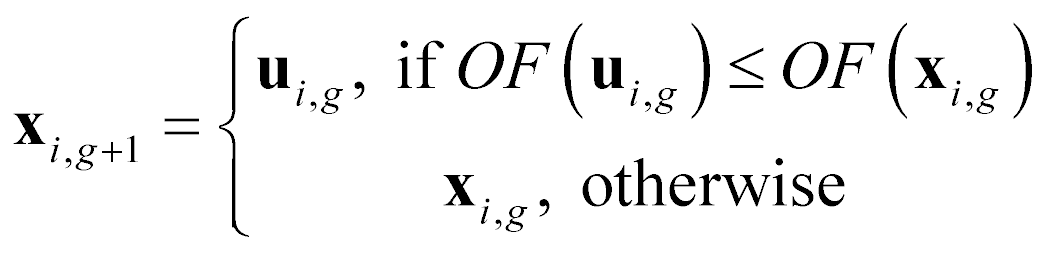The aforementioned formulation corresponds to the classic DE variant, denoted “rand/1/bin” , where “rand” indicates that base vectors are randomly chosen, “1” means that only one vector difference is used to form the mutated population, and the term “bin” (from binomial distribution) indicates that uniform crossover is employed during the formation of the trial population.

Another variant is denoted “best/1/bin” , where “best” indicates that the base vector used is the currently best vector in the population. Thus, the mutated population Pv,g is formed based on: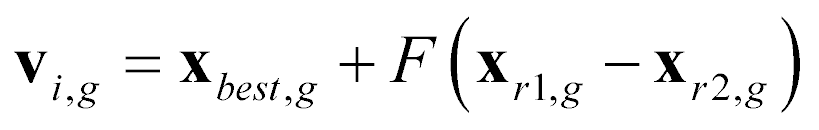In addition, “jitter” may be introduced to the parameter F and the previous equation is modified as follows: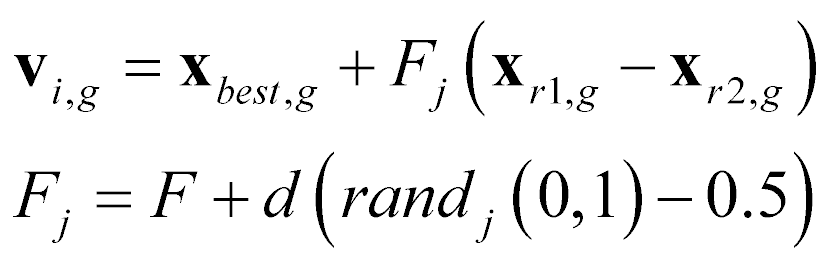where, randj is a random number with uniform distribution in (0,1) that is sampled anew each time and d is the magnitude of the jitter.

Another variant is available, denoted as “rand-best/1/bin”. This variant was presented in  and it is a mix of the “rand/1/bin” and “best/1/bin” variants. Define rb as the expected ratio of evaluations with “rand/1/bin” as opposed to the ones with “best/1/bin”. If randjrb, then evolution is carried out according to “rand/1/bin”. In the opposite case, “best/1/bin” is employed. Usual value of rb is 0.25. This variant was shown to combine the impressive initial performance of “best/1/bin” with the fine-tuning abilities of “rand/1/bin” .

Finally, a fourth variant is available, denoted as tourn/1/bin”. In this a tournament is employed for the selection of the base vector. Usually, a 2-member tournament works sufficiently.

Usual parameter values are the following: (a) p=30-50 vectors, (b) F=0.5, (c) Cr=0.9 (d) d=0.001 .

## Options in xlOptimizer

The following options are available in xlOptimizer:

#### Step 1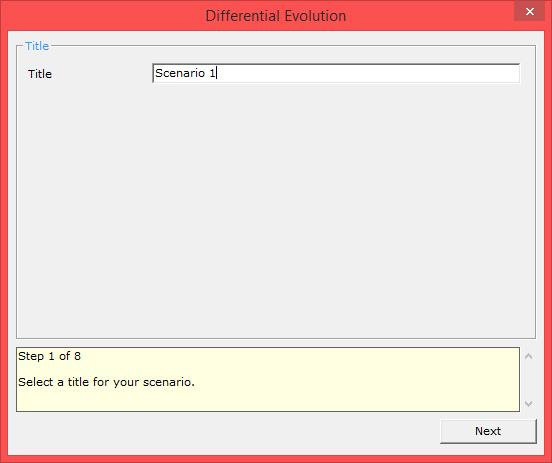Enter a short title for the scenario. The log files will be saved to a subfolder with this name.

#### Step 2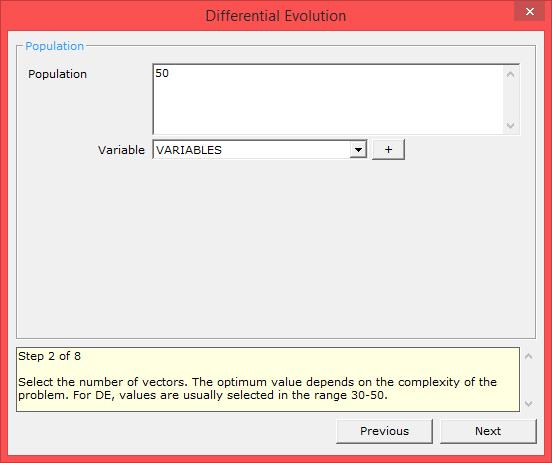Select the number of vectors. The optimum value depends on the complexity of the problem. For DE, values are usually selected in the range 30-50.

The variables that may be used in the expression are:

• VARIABLES: the number of variables.

#### Step 3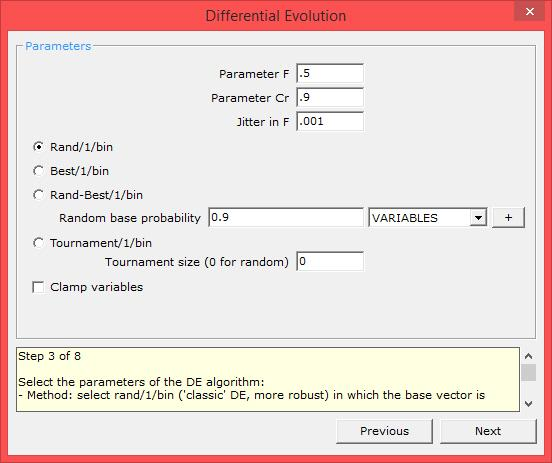Select the parameters of the DE algorithm:

• Parameter : used to scale the difference between two vectors to form a mutated vector according to v = x0 + F (x1 - x2) (for random base). Usual value is 0.5.
• Parameter C: used to form the trial vector based on the mutated vector. Usual value is 0.9.
• Jitter in F : used to introduce a jitter in parameter F . Usual value is 0.001 for all methods except for rand/1/bin, in which jitter can be equal to zero..
• Method: select one of the following:
• rand/1/bin ('classic' DE, more robust) in which the base vector is selected randomly,
• best/1/bin (faster, but less robust) in which the base vector is the current best vector of the population.
• rand-best/1/bin in which a predefined ratio of random based solutions are used. For random base probability rb = 0, the method is equivalent to best/1/bin, whereas for r= 1 it is equivalent to rand/1/bin. Usual values are 0.25 for easy problems, up to 0.9 for difficult problems.
• tourn/1/bin, in which a tournament of predefined size is used to select the base vector. Usually a 2-member tournament is sufficient.
• Clamp variables: if checked, the final vectors are clamped within the boundaries of each variable.

Step 4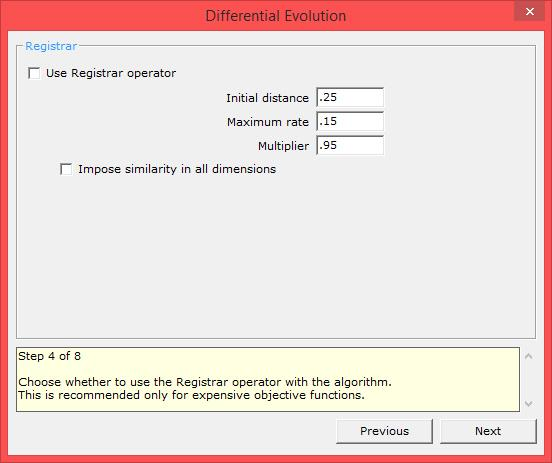Choose whether to use the Registrar operator with the algorithm. This is recommended only for expensive objective functions.

#### Step 5Select the termination criteria. At least one termination criterion must be active. Usually one selects a maximum number of function evaluations and/or a time limit.

#### Step 6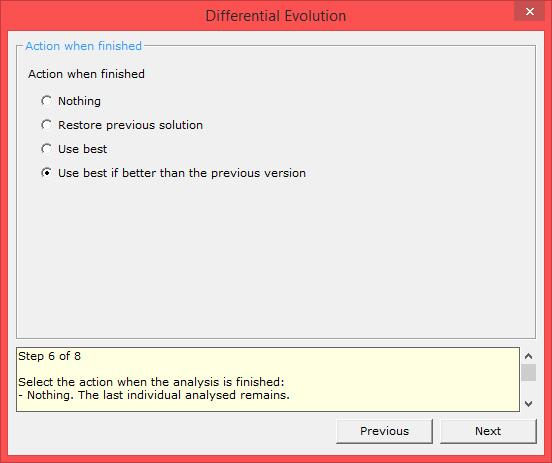Select the action when the analysis is finished:

• Nothing: The last individual analysed remains.
• Restore previous solution: The solution that was visible before the analysis is restored.
• Use best: The best solution that was discovered during the analysis is applied.
• Use best if better than the previous version (recommended): The best solution that was discovered during the analysis is applied only if it is better than the previous solution. In the opposite case the previous solution is restored.

#### Step 7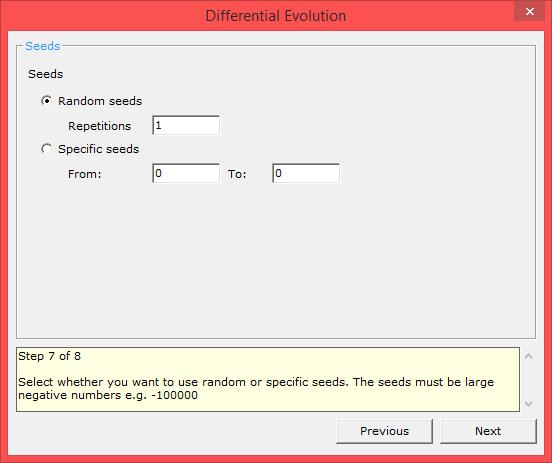Select whether you want to use random or specific seeds for the random number generator. The seeds must be large negative numbers e.g. -100000. Usually a number of analyses with random seeds is performed. The filename of the log is the seed used in the specific analysis.

#### Step 8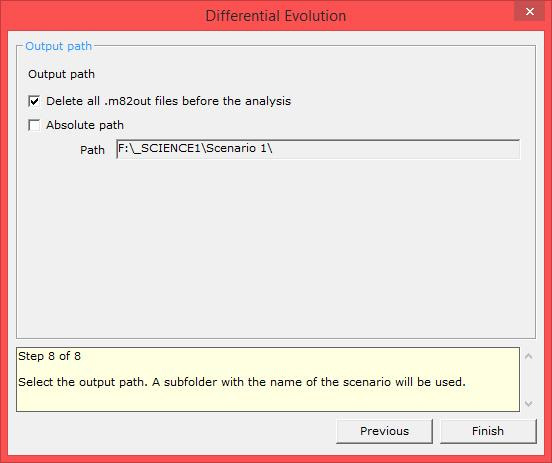Select the output path. A subfolder with the name of the scenario will be used. Usually a relative path is used i.e. the absolute path check box is not checked. This results in the output logs being saved in the same path as the project file.